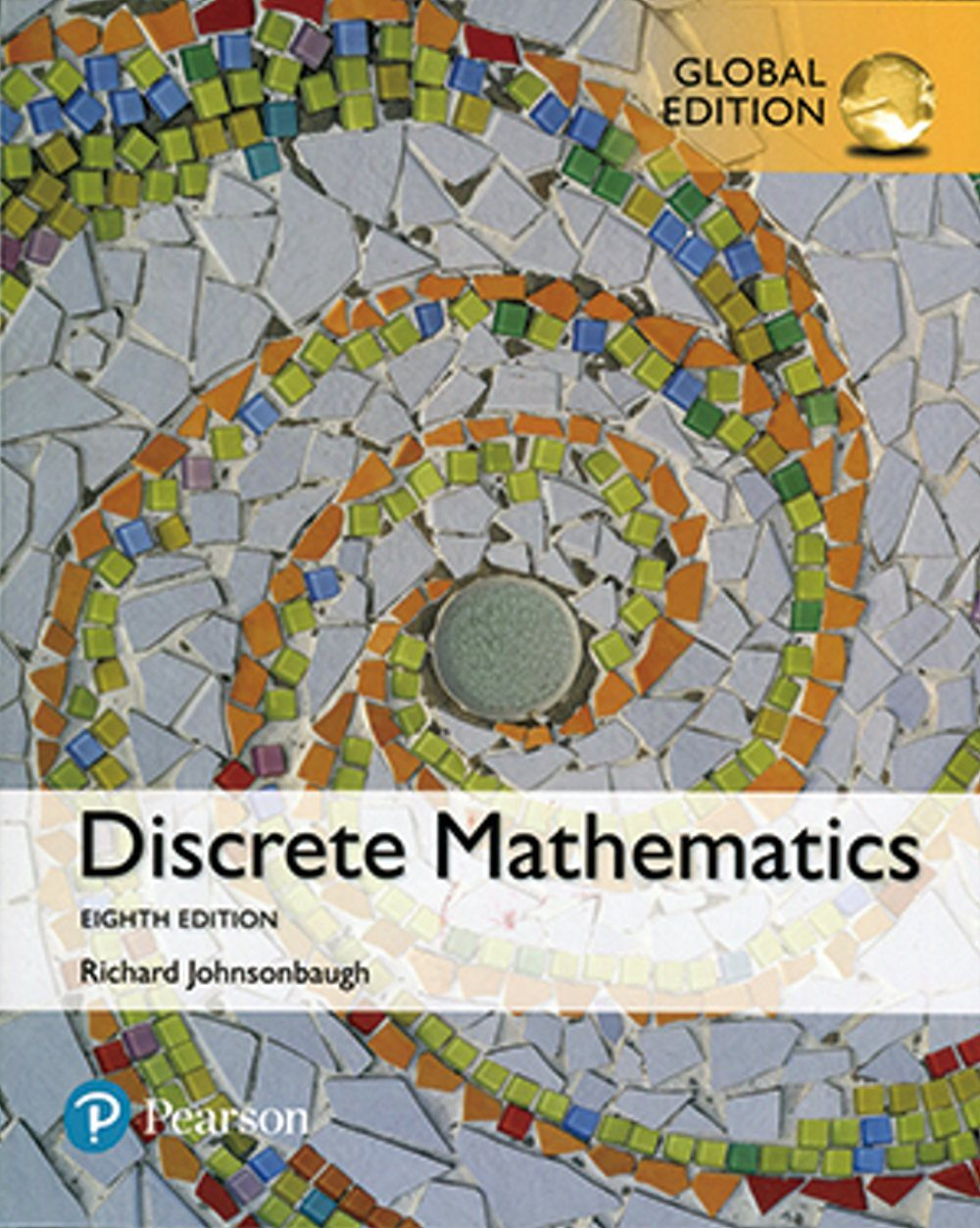{itemname} {itemname}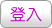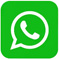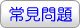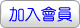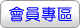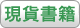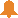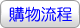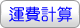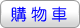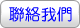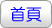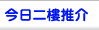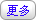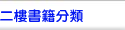Discrete Mathematics 7/e(PNIE)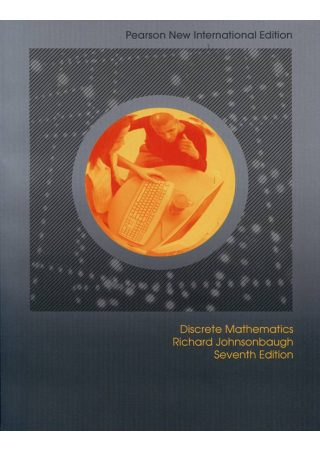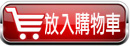沒有庫存 訂購需時10-14天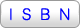9781292022611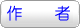Johnsonbaugh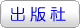華泰文化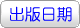2014年5月29日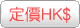367.00  元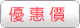HK\$ 348.65省下 \$18.35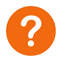ISBN：9781292022611
• 規格：平裝 / 750頁 / 普通級 / 單色印刷 / 7版
• 出版地：台灣

• [ 尚未分類 ]

For a one- or two-term introductory course in discrete mathematics.

Focused on helping students understand and construct proofs and expanding their mathematical maturity, this best-selling text is an accessible introduction to discrete mathematics. Johnsonbaugh’s algorithmic approach emphasizes problem-solving techniques. The Seventh Edition reflects user and reviewer feedback on both content and organization.

Features

1.Strong emphasis on reading and writing proofs – Illustrates most proofs of theorems with annotated figures to provide additional explanation and insight into the proofs.

2.Extensive discussion of algorithms, recursive algorithms, and the analysis of algorithms–The algorithms are written in a flexible form of pseudocode, which resembles currently popular languages such as C, C++, and Java.

3.Over 500 worked examples throughout the text.

4.Over 3500 exercises – Approximately one third have answers at the back of the book.

5.Extensive applications with an emphasis on computer science.

6.Emphasis on the interplay among the various topics.

7.Figures and tables – Illustrate concepts, show how algorithms work, elucidate proofs, and motivate the material. Figure captions provide additional explanation and insight into figures accompanying proofs.

8.Summaries of the mathematical and algorithm notation used in the book on the inside covers.

Ch 1 Sets and Logic

Ch 2 Proofs

Ch 3 Functions, Sequences, and Relations

Ch 4 Algorithms

Ch 5 Introduction to Number Theory

Ch 6 Counting Methods and The Pigeonhole Principle

Ch 7 Recurrence Relations

Ch 8 Graph Theory

Ch 9 Trees

Ch10 Network Models

Ch11 Boolean Algebras and Combinatorial Circuits

Ch12 Automata, Grammars, and Languages

 其 他 著 作 1. Discrete Mathematics (GE)(8版)